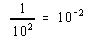Scientific Notation

Scientific Notation

Chemists often work with numbers that are extremely large or extremely small. For example, there are 10,300,000,000,000,000,000,000 carbon atoms in a 1-carat diamond each of which has a mass of 0.000,000,000,000,000,000,000,020 grams. It is impossible to multiply these numbers with most calculators because they can't accept either number as it is written here. To do a calculation like this, it is necessary to express these numbers in scientific notation, as a number between 1 and 10 multiplied by 10 raised to some exponent.

Exponent Review

Some of the basics of exponential mathematics are given below.

 Any number raised to the zero power is equal to 1. 1= 1 10= 1
 Any number raised to the first power is equal to itself. 11 = 1 101 = 10
 Any number raised to the nth power is equal to the product of that number times itself n-1 times. 22 = 2 x 2 = 4 105 = 10 x 10 x 10 x 10 x 10 = 100,000
 Dividing by a number raised to an exponent is the same as multiplying by that number raised to an exponent of the opposite sign.Converting to Scientific Notation

The following rule can be used to convert numbers into scientific notation: The exponent in scientific notation is equal to the number of times the decimal point must be moved to produce a number between 1 and 10. In 1990 the population of Chicago was 6,070,000 1000. To convert this number to scientific notation we move the decimal point to the left six times.

 6,070,000 = 6.070 x 106

To translate 10,300,000,000,000,000,000,000 carbon atoms into scientific notation, we move the decimal point to the left 22 times.

10,300,000,000,000,000,000,000 = 1.03 x 1022

To convert numbers smaller than 1 into scientific notation, we have to move the decimal point to the right. The decimal point in 0.000985, for example, must be moved to the right four times.

0.000985 = 9.85 x 10-4

Converting 0.000,000,000,000,000,000,000,020 grams per carbon atom into scientific notation involves moving the decimal point to the right 23 times.

0.000,000,000,000,000,000,000,020 = 2.0 x 10-23

The primary reason for converting numbers into scientific notation is to make calculations with unusually large or small numbers less cumbersome. Because zeros are no longer used to set the decimal point, all of the digits in a number in scientific notation are significant, as shown by the following examples.

 2.4 x 1022 2 significant figures 9.80 x 10-4 3 significant figures 1.055 x 10-22 4 significant figures

 Practice Problem 8  Convert the following numbers into scientific notation. (a) 0.004694 (b) 1.98 (c) 4,679,000 Click here to check your answers to Practice Problem 8